# Fast AI Machine Learning (Lessons 1–2)

Introduction to Random Forests

This first lesson let us know the basic concepts of Machine Learning, through understanding what is a “Random Forest” algorithm. Concepts of the use of Google Collaboratory were also introduced. This is a virtual space where Python 2.0 or Python 3.0 code can be executed, this type of IDE is provided by the Jupyter Notebook environment.

Step 1. Prepare the environment for the execution of the Random Forest algorithm. For this, it is necessary to have a google account in order to have access to the collaborative work environment, the link is: https://colab.research.google.com/, once you have logged in to your Google account.

Figure 1 shows the created virtual machines, or the option to create a new Python notebook with versions 2.0 or 3.0.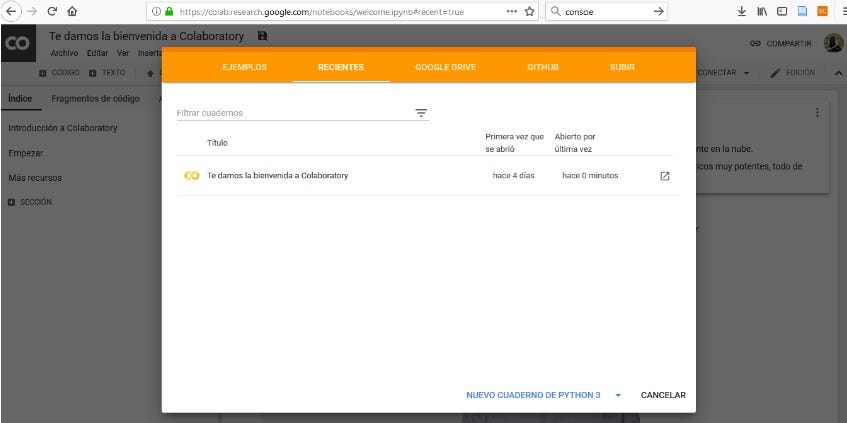Figure 1. Google Collaborative Start window.

The created workspace is called “notebook”, which enables a virtual machine ready to execute what is required, in this case some of the following dependencies will be installed in Python 3.0.

Among the first things we will need are the libraries used by FastAI, for this we access the package manager to the FastAI repository on Github.

The previous command is executed by pressing the “run” button

Once the command has been runned, we can see that all the required packages were successfully installed (Figure 2).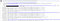Figure 2.

To create a new cell, we use the button

, now we can write more lines of code and execute them separately.

There are several tools like Google Colabs, for example the environment provided by Amazon, AWS or Amazon web Services that can be found through the following link: https://docs.aws.amazon.com/dlami/latest/devguide/setup-jupyter.html.

Once all the libraries and/or FastAI modules have been loaded into the environment, it is necessary to execute the autoreload command, in order to automatically reload the modules without rebooting the system (Figure 3).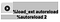Figure 3.

Additionally, we need to load the Matplotlib library that allows multiplatform data visualization in NumPy matrices (Figure 4).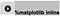Figure 4.

Other libraries that need to be imported are: pandas for data management, fastai and sklearn that facilitate working with machine learning (Figure 5).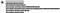Figure 5.

Step 2. Once the environment to work with “Random Forest” is ready, it will be necessary to load the data set in the Google Colaboratory notebook. It is a good idea to have the data set already in the cloud (e.g. Google Drive) to make the data importation to the work environment simpler. Figure 6 shows the line of code that let us work in an integrated way between Google Colaboratory and Gdrive.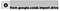Figure 6.

The result of the previous instruction is shown in Figure 7, where we need to obtain an authorization code to perform the requested action. For this, we need to access the given URL and copy the code as shown in Figure 8, so that the data set can be mounted into the virtual work machine.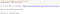Figura 7.

Figura 7.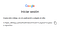Figura 8.

Figura 8.

After this, the contents of our Gdrive containing the mounted data set can be accessed, here the data set folder is called “datos” (Figure 9).Figure 9.

The result will be the name of the data set elements as shown in Figure 10.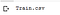Figure 10.

Once the built-in data set has been identified, some of the data contained in the data set can be displayed in order to verify the data by running the following cell (Figure 11).Figure11.

The expected result will be as in Figure 12, where all the contained data loaded into the virtual machine can be displayed.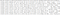Figure 12.

Step 3. Once the data has been loaded into the Colaboratory Notebook virtual machine, a Pandas dataframe must be used to store the data contained in the loaded dataset, this can be done by using the following command (Figure 13):Figure 13.

The PATH variable contains the system location where the data set is stored within the virtual machine (Figure 14).Figure 14.

To verify that the data loaded into the dataframe are correct, a part of it is displayed with a function that shows a fragment of the loaded data set, in this case we define to display 1000 rows and 1000 columns by using the following code (Figure 15):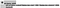Figure 15.

With the following line of code (Figure 16), the defined function “display_all” is executed and the result will be as shown in figure 17.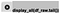Figure 16.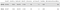Figure 17.

Step 4. Once the data has been loaded into the Colaboratory Notebook virtual machine, we are ready to start programming by using the machine learning libraries.

At this point it is necessary to remember that a Random Forest is a technique based on decision trees that let us know the importance of each variable. The target variable in a Random Forest can be categorical or quantitative, and the set of explanatory variables also (Figure 18 in spanish).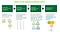Figure 18.

Then, we’ll use the function “RandomForestRegressor”, which allows estimating the number of groups to create, adjusting to the classification sub-samples. This function receives several parameters, the one we have used indicates that all the available processors are available for its execution (Figure 19).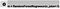Figure 19.

With the following instruction (Figure 20), we indicate the algorithm what the target variable is and it is separated from the data set for the prediction.Figure 20.

The execution of the previous instruction, returns an error because the data set should be a set of numbers, by running the command dtypes we can see that there are columns of different types and not only numeric.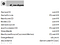Figure 21.

At this point it is important to review how dataframes work for understanding the following properties, we suggest this link: https://pandas.pydata.org/pandas-docs/stable/reference/api/pandas.DataFrame.dtypes.html

Step 5. Once the column or columns that should contain another type of data have been identified, they are separated, in this case we work with the fields: SalesDate and UsageBand.

Salesdate is a continuous variable, however, for the analysis its continuity is not relevant in detail and it will suffice to obtain a column with the years, for this an object named fld is created using the following command (Figure 22).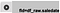Figure 22.

The attributes of the just created fld object can be seen with the dt property (Figure 23).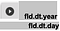Figure 23.

To finish this step, several fields are created with the required date information, for this we use the function add_datepart (Figure 24).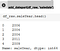Figure 24.

Step 6. On the other hand, the UsageBand field will be categorized as ‘High’, ‘Medium’, and ‘Low’, since for the analysis the field is not required to be a continuous variable, but to classify by ranks or groups. Categorization allows saving calculation if the categories are well chosen. To do this, the commands in Figure 25 are executed: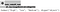Figure 25.

An interesting fact is that the NaN values are not categorized and get assigned with -1.

For the categorization to be applied to the column, the command shown in Figure 26 is executed.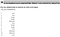Figure 26.

Once categorized the column would look like Figure 27:Figure 27.

ML section redacted by Martha San Andrés.

DL section redacted by David Vivas.

ML and DL sections translated and edited by David Francisco Dávila Ortega, MSc. — Eng.

Review by Paolo Paste

bootcampai.org